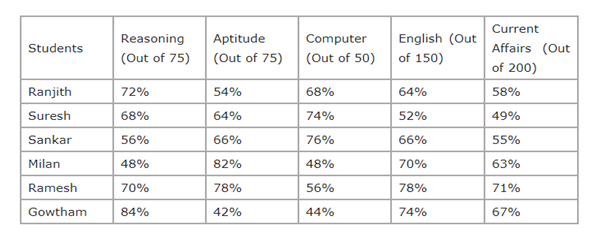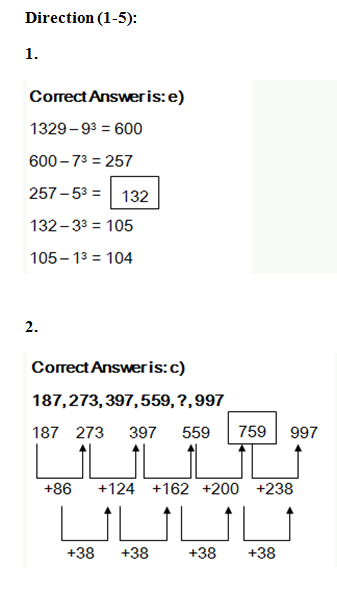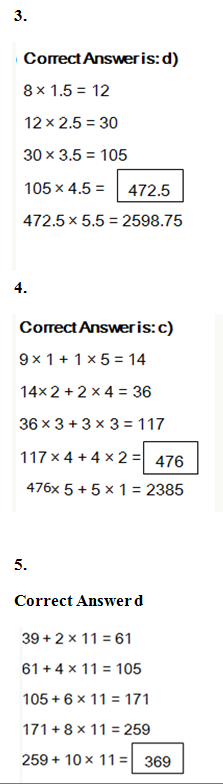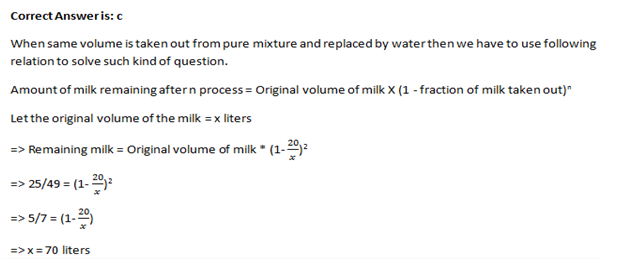# “20-20” Quantitative Aptitude | Crack SBI Clerk 2018 Day-11

Dear Friends, SBI Clerk 2018 Notification has been released we hope you all have started your preparation. Here we have started New Series of Practice Materials specially for SBI Clerk 2018. Aspirants those who are preparing for the exams can use this “20-20” Quantitative Aptitude Questions.

[WpProQuiz 1178]

Click “Start Quiz” to attend these Questions and view Explanation

### Click Here for SBI Clerk Prelims and Mains 2018 – Full Length Mock Test

Direction (1-5): The following series are based on a specific pattern. Find the next number following the pattern.

1. 1329, 600, 257, ? , 105, 104
1. 128
2. 130
3. 134
4. 126
5. None of these
1. 187, 273, 397, 559, ?, 997
1. 899
2. 814
3. 759
4. 748
5. None of these
1. 8, 12, 30, 105, ? , 2598.75
1. 420
2. 470
3. 422.5
4. 472.5
5. None of these
1. 9, 14, 36, 117,?, 2385
1. 516
2. 826
3. 476
4. 626
5. None of these
1. 39, 61, 105, 171, 259, ?
1. 360
2. 379
3. 386
4. 369
5. None of these

Directions (Q. 6-10): What approximate value should come in place of question mark in the following questions? (you are not expected to calculate the exact value)

6). [(√530 × 36.003) ÷ 47.987] × ? = 5863.10376

1. 640
2. 750
3. 340
4. 360
5. 680

7). (77.987 % of 358) + (68.55% of 729) = ?

1. 780
2. 705
3. 840
4. 825
5. 695

8). √624.995 + (4.9989)^2 = ? ÷ (1 / 4.9900865)

1. 18
2. 34
3. 44
4. 10
5. 25

9). 989.001 + 1.00982 × 76.792 = ?

1. 1150
2. 1066
3. 1240
4. 1188
5. 1044

10). 63.9872 × 9449.8780 ÷ 243.003 = (?)2

1. 60
2. 75
3. 90
4. 40
5. 50
1. A can complete a piece of work in 8 days while B can complete the same work in 12 days. They work together for 3 days. Then A quits the work. In how many days will B now be able to finish the remaining work?
1. 3 days
2. 5.2 days
3. 4.5 days
4. 2.4 days
5. 3.4 days
1. Rakesh Gangwal and Rahul Bhatia invested in a business in the ratio 10:13. What is the total profit, if Rahul Bhatia’s profit share is Rs.832 and 8% of the total profit goes to charity?
1. Rs.1400
2. Rs.1500
3. Rs.1600
4. Rs.1700
5. Rs.1800
1. The amount obtained on Rs. 24000 at an interest 5% compounded annually for certain period of time is Rs 27,783. Then find the time in years?
1. 2 years
2. 3 years
3. 4 years
4. 5 years
5. 1 year
1. From a Jar containing pure milk, 20 liters is taken out and is replaced with water. This process is carried twice and at the end of the process the ratio of milk to water in the Jar becomes 25:24. What was the original volume of the pure milk?
1. 60 liters
2. 45 liters
3. 70 liters
4. 75 liters
5. None of these
1. 72% of the employees of a certain ministry choose HRA according to 7th CPC and 44% choose to draw old pension scheme. If each employee selected at least one option from HRA or old pension scheme and 40 took both, then the total number of employees in the ministry is:
1. 200
2. 250
3. 240
4. 300
5. None of these

Directions (Q. 16-20): The following table shows the percentage of marks obtained by six students in five different subjects. Answer the following questions based on this table.1. What is the total mark scored by Sankar in all the subjects together?
1. 352.5
2. 312.5
3. 348.5
4. 327.5
5. 338.5
1. What is the difference between the total marks obtained by Milan in Aptitude and English and that obtained by Sankar in the same subject?
1. 22
2. 17
3. 18
4. 12
5. 15
1. The marks scored by Ranjith in Reasoning is approximately what per cent of the marks scored by him in English?
1. 60%
2. 52%
3. 50%
4. 56%
5. 63%
1. What is the average mark scored by all the students in Reasoning?
1. 49.75
2. 43.25
3. 50.50
4. 52.75
5. 45.75
1. What is the overall percentage of marks scored by Suresh? (Answer in approximate value.)
1. 53%
2. 57%
3. 61%
4. 63%
5. 51%Directions (Q. 6-10):

[( √530 x 36.003) ÷ 47.987] x ? = 5863.10376

? = 5863 / [( √529 x 36) ÷ 48] = 5863 / [(23 × 36) ÷ 48] = 340

? = (77.987% of 358) + (68.55% of 729)

? = [(78/100) × 358] + [(69/100) × 725]

= 280 + 500 = 780

√624.995 + (4.9989)^2

= ? ÷ (1 / 4.9900865)

or, √625 + (5)2= ? ÷ (1/5)

? = 1/5 (25 + 25) = 10

? = 989.001 + 1.00982 × 76.792

= 990 + 1 x 76 = 1066

(?)2= 63.9872 x 9449.8780 ÷ 243.0034

= 64 x 9450 ÷ 243 = 64 x 39 = 2496

Now, (?)2 = 2496

? = 50

A alone completes the work in 8 days. So, he does 1/8th of the work in 1 day.

B alone completes the work in 12 days. So, he does 1/12th of the work on 1 day.

Hence, total work completed together in 1 day

= (1/8) + (1/12) = 5/24

A and B work together for only 3 days.

Work completed together in 3 days = 3*(5/24) = 15/24

Amount of work that B has to complete alone = 1 – (15/24) = 9/24

Number of days that B will take to complete the work = (9/24) / (1/12) = 4.5 days

Hence, B can finish the remaining work in 4.5 days.

Total profit = (832/13)*23*(100/92)=1600

We know that, A = P (1+R/100)T

27,783 = 24,000 (1+5/100)T

(21/20)T=9261/8000

(21/20)T=(21/20)3

T = 3 years

Hence the required time = 3 years

14.=> Percentage of employees who choose HRA = 72%

=> Percentage of employees who choose Old Pension Scheme = 44%

=> Percentage of employees who selected both = 72+44-100

=16%

=> Which is equal to 40 (given)

=> Therefore, total employees = 40*100/16

=250

Directions (Q. 16-20):

Total marks scored by Sankar in all the subjects together

= 75 × 0.56 + 75 × 0.66 + 200 × 0.55 + 50 × 0.76 + 150 × 0.66

= 42 + 49.5 + 110 + 38 + 99

= 338.5

Total marks obtained by Milan

= (75 ×0.82) + (150 × 0.70)

= 61.5 + 105

= 166.5

Total marks obtained by Sankar

= (75 × 0.66) + (150 + 0.66)

= 49.5 + 99

= 148.5

Difference = 166.5 – 148.5 = 18

Marks scored by Ranjith in Reasoning

= 75 × 0.72 = 54

Marks scored by Ranjith in English

= 150 × 0.64 = 96

Required % = 54/96 × 100

= 56.25%

= 56%

Average marks of all students in Reasoning

= 1/6 [75 × (0.84 + 0.68 + 0.72 + 0.48 + 0.70 + 0.56)]

= 1/6 [75 × 3.98]

Average = 298.5/6

= 49.75

Marks scored by Suresh

= 75 × 0.68 + 75 × 0.64 + 200 × 0.49 + 50 × 0.74 + 150 × 0.52

= 51 +48 + 98 + 37 + 78=312

Required % = 312/550 × 100

= 56.72%

= 57%